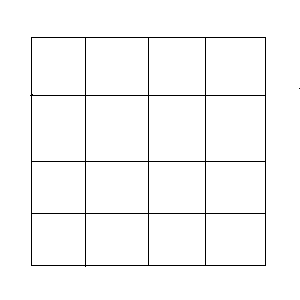You may also likeConsecutive Numbers

An investigation involving adding and subtracting sets of consecutive numbers. Lots to find out, lots to explore.Calendar Capers

Choose any three by three square of dates on a calendar page...Days and Dates

Investigate how you can work out what day of the week your birthday will be on next year, and the year after...

Distinct Diagonals

Age 11 to 14 ShortChallenge Level

Sixteen unit squares are arranged to form a square array as shown in the diagram.What is the maximum number of diagonals that can be drawn in these unit squares so that no two diagonals share a common point (including endpoints)?

If you liked this problem, here is an NRICH task which challenges you to use similar mathematical ideas.

This problem is taken from the UKMT Mathematical Challenges.
You can find more short problems, arranged by curriculum topic, in our short problems collection.